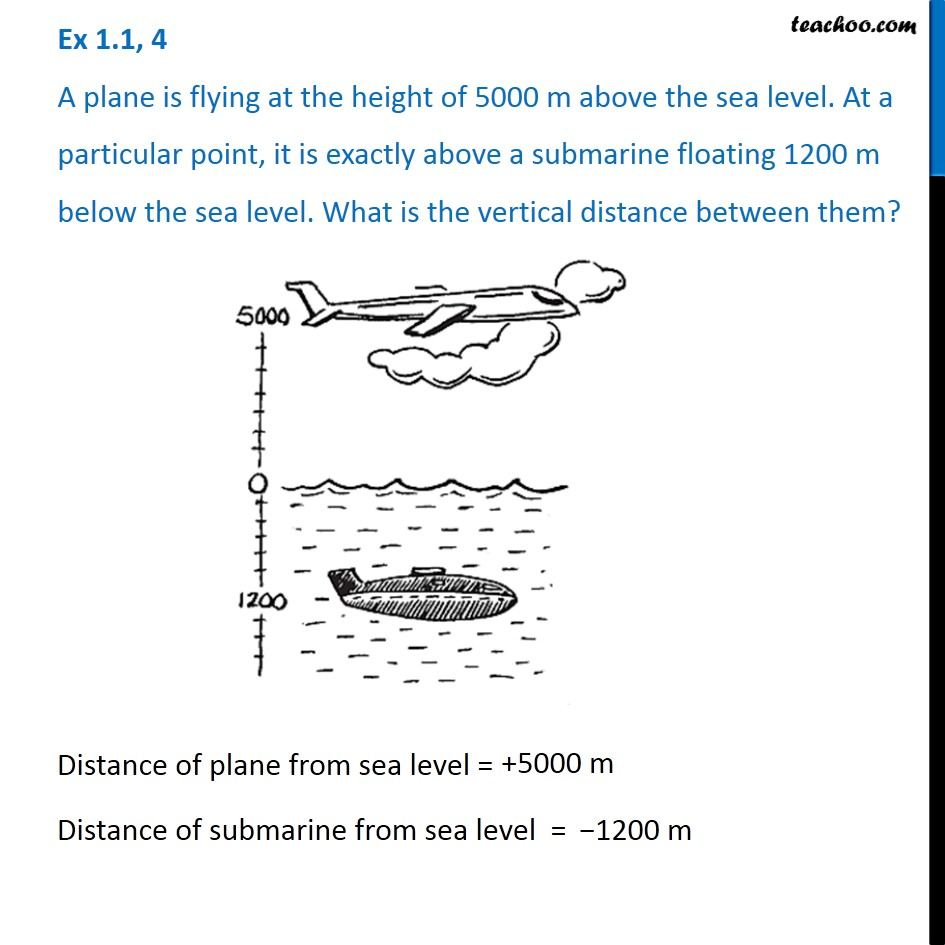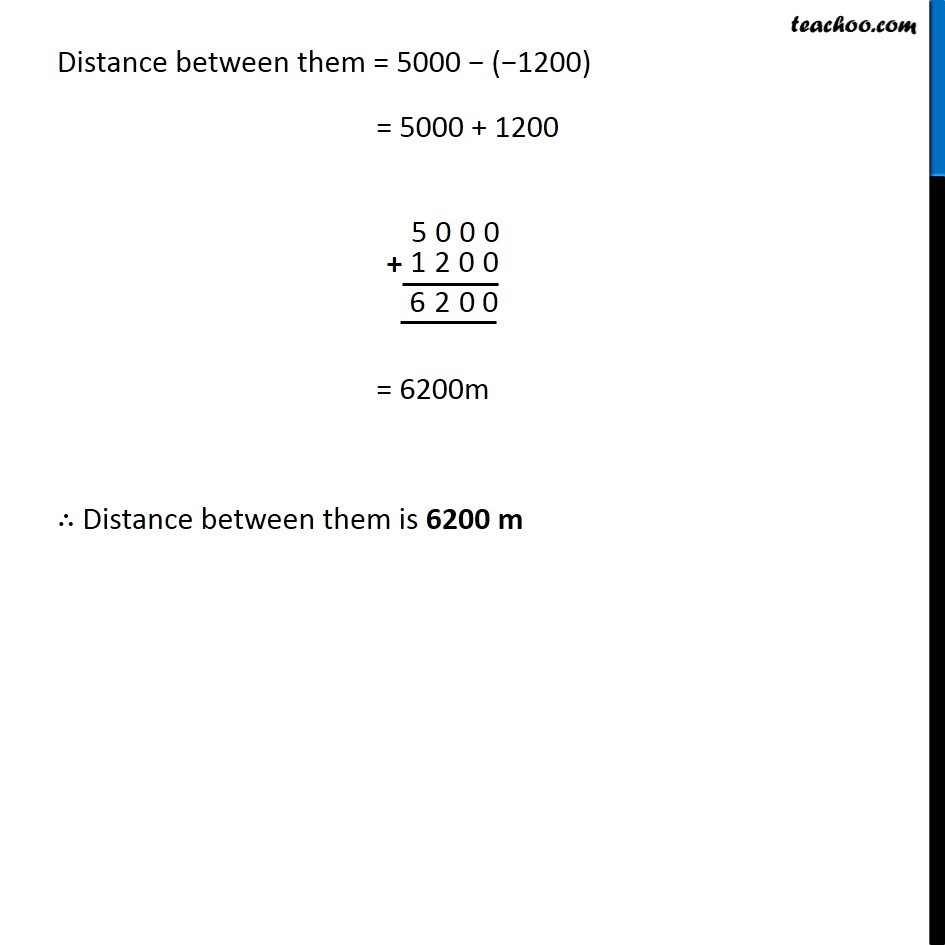Chapter 1 Class 7 Integers
Concept wiseLearn in your speed, with individual attention - Teachoo Maths 1-on-1 Class

### Transcript

Question 4 A plane is flying at the height of 5000 m above the sea level. At a particular point, it is exactly above a submarine floating 1200 m below the sea level. What is the vertical distance between them? Distance of plane from sea level = +5000 m Distance of submarine from sea level = −1200 m Distance between them = 5000 − (−1200) = 5000 + 1200 = 6200m ∴ Distance between them is 6200 m